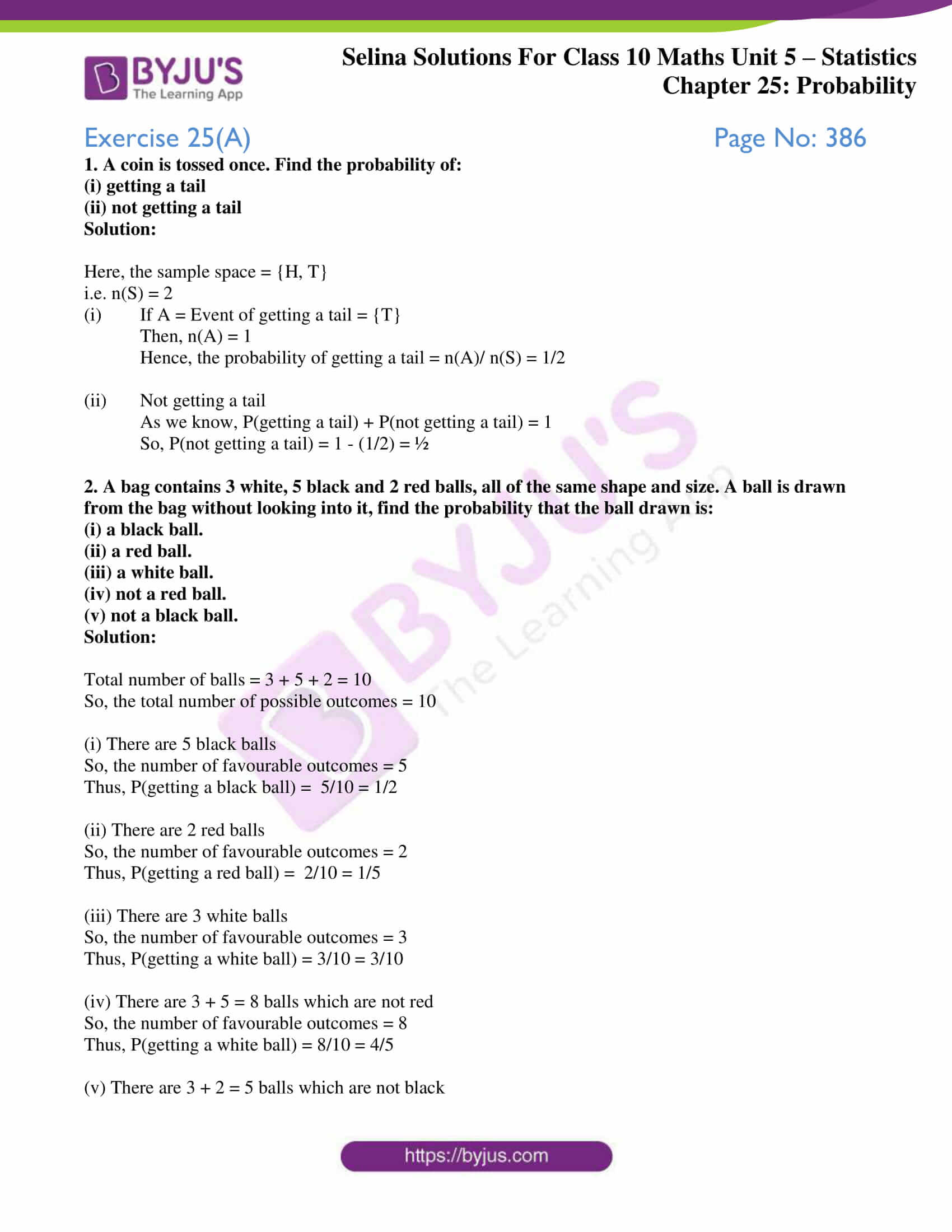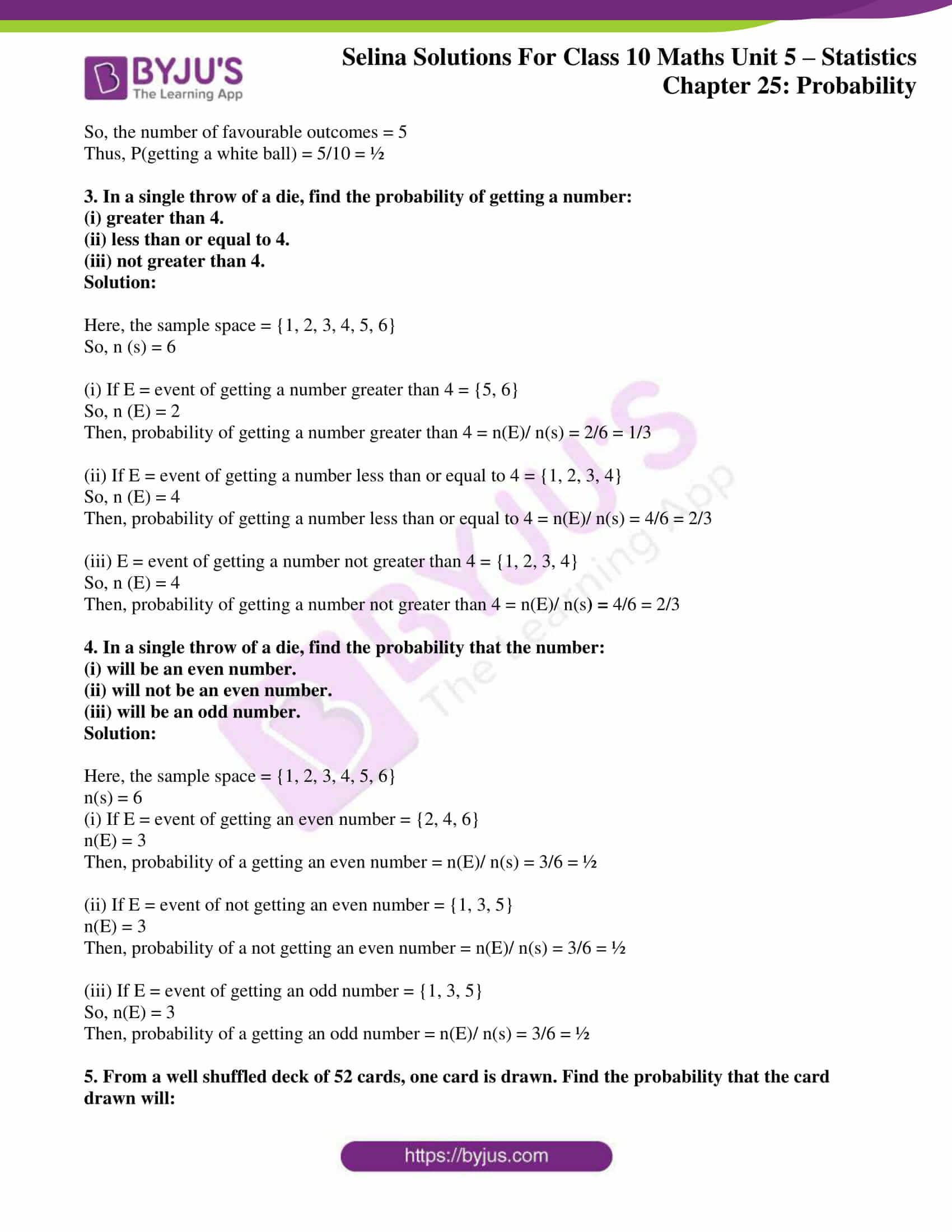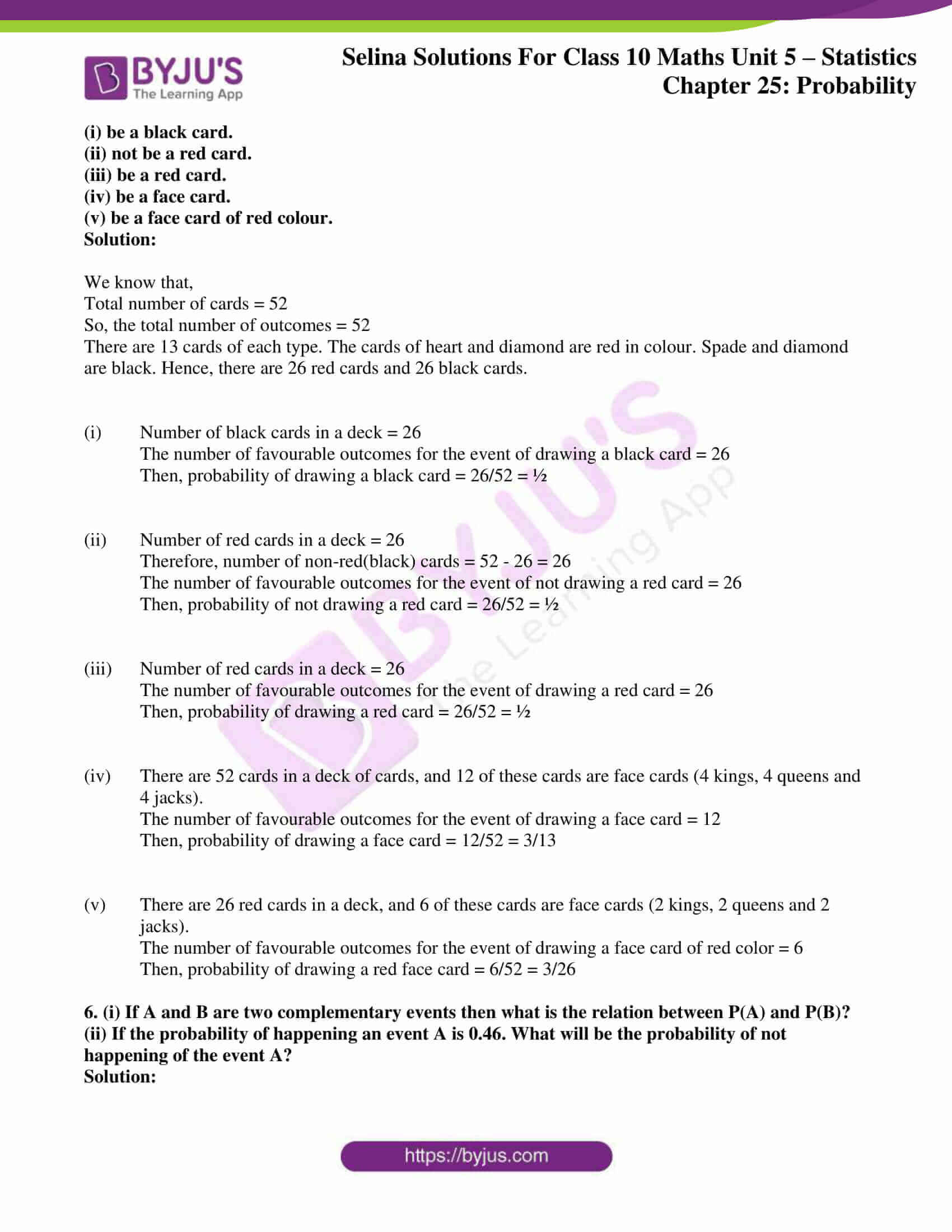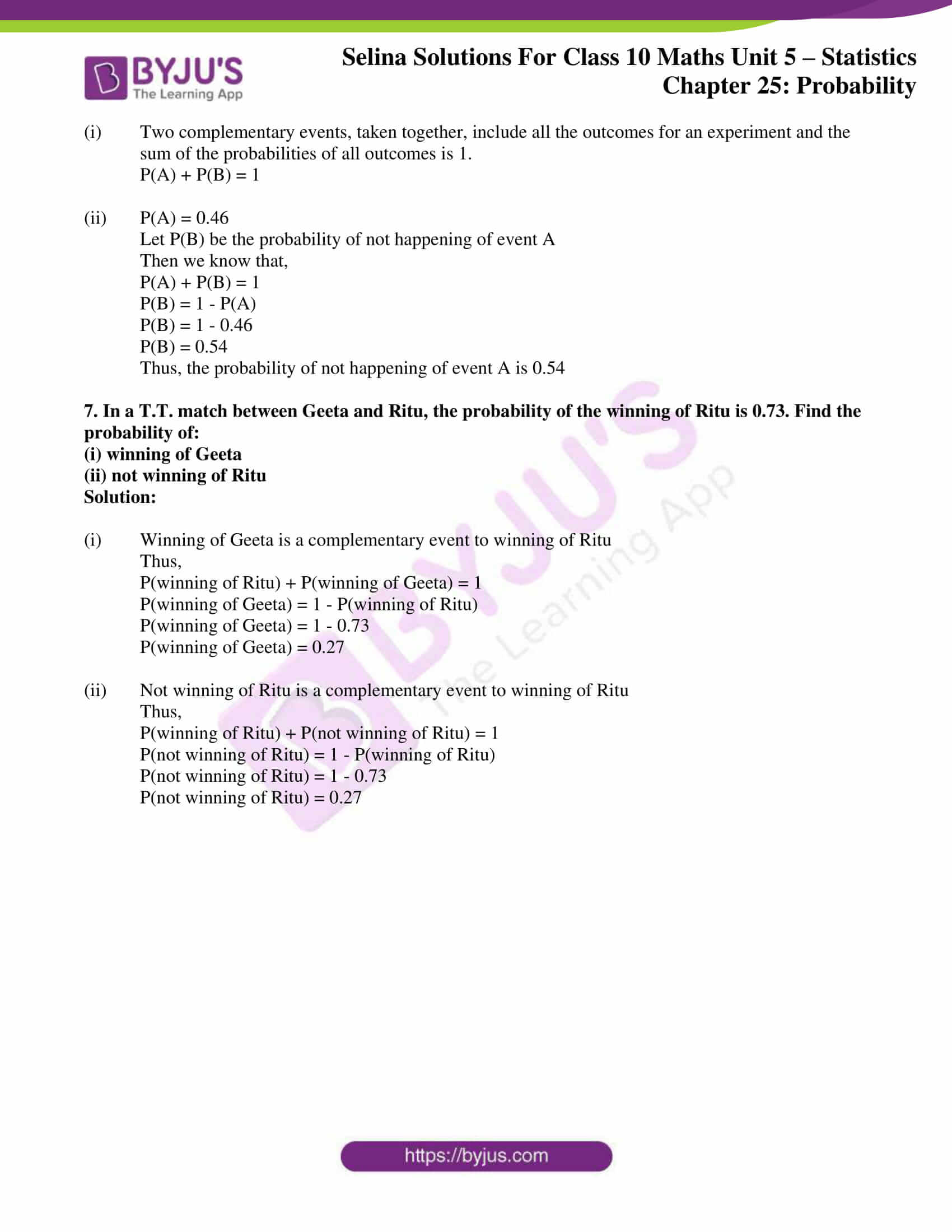The measure of probability by empirical and classical methods are discussed in this exercise. The Selina Solutions for Class 10 Maths is a one-stop solution to clarify doubts when solving problems of this chapter and others. The solutions are created as per the latest ICSE marking schemes. The solutions of this exercise are available in the Concise Selina Solutions for Class 10 Maths Chapter 25 Probability Exercise 25(A) PDF, provided in the link given below.

## Selina Solutions Concise Maths Class 10 Chapter 25 Probability Exercise 25(A) Download PDF### Access Selina Solutions Concise Maths Class 10 Chapter 25 Probability Exercise 25(A)

1. A coin is tossed once. Find the probability of:

(i) getting a tail

(ii) not getting a tail

Solution:

Here, the sample space = {H, T}

i.e. n(S) = 2

(i) If A = Event of getting a tail = {T}

Then, n(A) = 1

Hence, the probability of getting a tail = n(A)/ n(S) = 1/2

(ii) Not getting a tail

As we know, P(getting a tail) + P(not getting a tail) = 1

So, P(not getting a tail) = 1 – (1/2) = ½

2. A bag contains 3 white, 5 black and 2 red balls, all of the same shape and size. A ball is drawn from the bag without looking into it, find the probability that the ball drawn is:

(i) a black ball.

(ii) a red ball.

(iii) a white ball.

(iv) not a red ball.

(v) not a black ball.

Solution:

Total number of balls = 3 + 5 + 2 = 10

So, the total number of possible outcomes = 10

(i) There are 5 black balls

So, the number of favourable outcomes = 5

Thus, P(getting a black ball) =  5/10 = 1/2

(ii) There are 2 red balls

So, the number of favourable outcomes = 2

Thus, P(getting a red ball) =  2/10 = 1/5

(iii) There are 3 white balls

So, the number of favourable outcomes = 3

Thus, P(getting a white ball) = 3/10 = 3/10

(iv) There are 3 + 5 = 8 balls which are not red

So, the number of favourable outcomes = 8

Thus, P(getting a white ball) = 8/10 = 4/5

(v) There are 3 + 2 = 5 balls which are not black

So, the number of favourable outcomes = 5

Thus, P(getting a white ball) = 5/10 = ½

3. In a single throw of a die, find the probability of getting a number:

(i) greater than 4.

(ii) less than or equal to 4.

(iii) not greater than 4.

Solution:

Here, the sample space = {1, 2, 3, 4, 5, 6}

So, n (s) = 6

(i) If E = event of getting a number greater than 4 = {5, 6}

So, n (E) = 2

Then, probability of getting a number greater than 4 = n(E)/ n(s) = 2/6 = 1/3

(ii) If E = event of getting a number less than or equal to 4 = {1, 2, 3, 4}

So, n (E) = 4

Then, probability of getting a number less than or equal to 4 = n(E)/ n(s) = 4/6 = 2/3

(iii) E = event of getting a number not greater than 4 = {1, 2, 3, 4}

So, n (E) = 4

Then, probability of getting a number not greater than 4 = n(E)/ n(s) = 4/6 = 2/3

4. In a single throw of a die, find the probability that the number:

(i) will be an even number.

(ii) will not be an even number.

(iii) will be an odd number.

Solution:

Here, the sample space = {1, 2, 3, 4, 5, 6}

n(s) = 6

(i) If E = event of getting an even number = {2, 4, 6}

n(E) = 3

Then, probability of a getting an even number = n(E)/ n(s) = 3/6 = ½

(ii) If E = event of not getting an even number = {1, 3, 5}

n(E) = 3

Then, probability of a not getting an even number = n(E)/ n(s) = 3/6 = ½

(iii) If E = event of getting an odd number = {1, 3, 5}

So, n(E) = 3

Then, probability of a getting an odd number = n(E)/ n(s) = 3/6 = ½

5. From a well shuffled deck of 52 cards, one card is drawn. Find the probability that the card drawn will:

(i) be a black card.

(ii) not be a red card.

(iii) be a red card.

(iv) be a face card.

(v) be a face card of red colour.

Solution:

We know that,

Total number of cards = 52

So, the total number of outcomes = 52

There are 13 cards of each type. The cards of heart and diamond are red in colour. Spade and diamond are black. Hence, there are 26 red cards and 26 black cards.

(i) Number of black cards in a deck = 26

The number of favourable outcomes for the event of drawing a black card = 26

Then, probability of drawing a black card = 26/52 = ½

(ii) Number of red cards in a deck = 26

Therefore, number of non-red(black) cards = 52 – 26 = 26

The number of favourable outcomes for the event of not drawing a red card = 26

Then, probability of not drawing a red card = 26/52 = ½

(iii) Number of red cards in a deck = 26

The number of favourable outcomes for the event of drawing a red card = 26

Then, probability of drawing a red card = 26/52 = ½

(iv) There are 52 cards in a deck of cards, and 12 of these cards are face cards (4 kings, 4 queens and 4 jacks).

The number of favourable outcomes for the event of drawing a face card = 12

Then, probability of drawing a face card = 12/52 = 3/13

(v) There are 26 red cards in a deck, and 6 of these cards are face cards (2 kings, 2 queens and 2 jacks).

The number of favourable outcomes for the event of drawing a face card of red color = 6

Then, probability of drawing a red face card = 6/52 = 3/26

6. (i) If A and B are two complementary events then what is the relation between P(A) and P(B)?

(ii) If the probability of happening an event A is 0.46. What will be the probability of not happening of the event A?

Solution:

(i) Two complementary events, taken together, include all the outcomes for an experiment and the sum of the probabilities of all outcomes is 1.

P(A) + P(B) = 1

(ii) P(A) = 0.46

Let P(B) be the probability of not happening of event A

Then we know that,

P(A) + P(B) = 1

P(B) = 1 – P(A)

P(B) = 1 – 0.46

P(B) = 0.54

Thus, the probability of not happening of event A is 0.54

7. In a T.T. match between Geeta and Ritu, the probability of the winning of Ritu is 0.73. Find the probability of:

(i) winning of Geeta

(ii) not winning of Ritu

Solution:

(i) Winning of Geeta is a complementary event to winning of Ritu

Thus,

P(winning of Ritu) + P(winning of Geeta) = 1

P(winning of Geeta) = 1 – P(winning of Ritu)

P(winning of Geeta) = 1 – 0.73

P(winning of Geeta) = 0.27

(ii)  Not winning of Ritu is a complementary event to winning of Ritu

Thus,

P(winning of Ritu) + P(not winning of Ritu) = 1

P(not winning of Ritu) = 1 – P(winning of Ritu)

P(not winning of Ritu) = 1 – 0.73

P(not winning of Ritu) = 0.27